Home > CCA2 > Chapter 9 > Lesson 9.3.3 > Problem9-108

9-108.
1. Solve each equation. Homework Help ✎

1.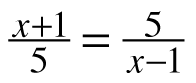2.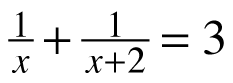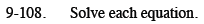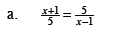Multiply both sides by 5(x − 1) to cancel the denominators.

$x=\pm\sqrt{26}$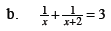Multiply both sides by x(x + 2).

You will need to use the Quadratic Formula to get the answer.